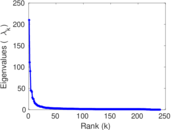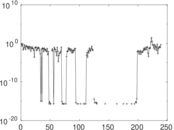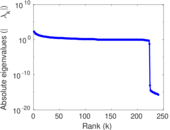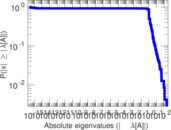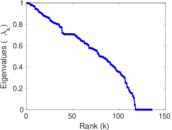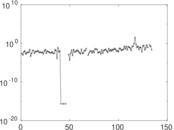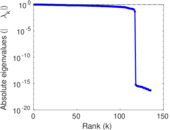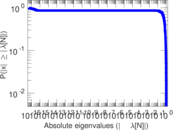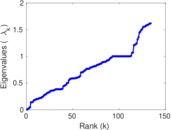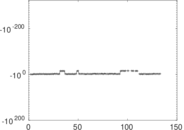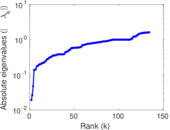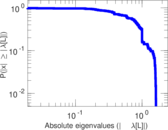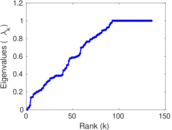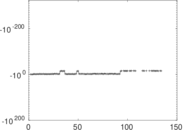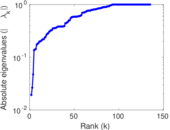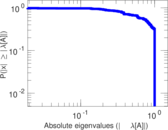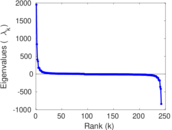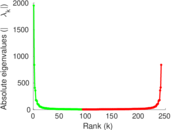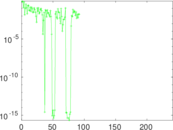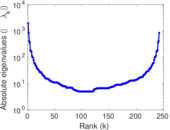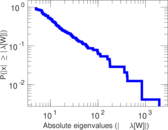# Wikibooks edits (hy)

This is the bipartite edit network of the Armenian Wikibooks. It contains users and pages from the Armenian Wikibooks, connected by edit events. Each edge represents an edit. The dataset includes the timestamp of each edit.

 Code `bhy` Internal name `edit-hywikibooks` Name Wikibooks edits (hy) Data source http://dumps.wikimedia.org/ AvailabilityDataset is available for download Consistency checkDataset passed all tests Category Authorship network Dataset timestamp 2017-10-20 Node meaning User, article Edge meaning Edit Network formatBipartite, undirected Edge typeUnweighted, multiple edges Temporal dataEdges are annotated with timestamps

## Statistics

 Size n = 1,956 Left size n1 = 242 Right size n2 = 1,714 Volume m = 4,927 Unique edge count m̿ = 2,379 Wedge count s = 333,359 Claw count z = 59,977,339 Cross count x = 9,407,216,590 Square count q = 28,617 4-Tour count T4 = 1,569,154 Maximum degree dmax = 1,760 Maximum left degree d1max = 1,760 Maximum right degree d2max = 211 Average degree d = 5.037 83 Average left degree d1 = 20.359 5 Average right degree d2 = 2.874 56 Fill p = 0.005 735 46 Average edge multiplicity m̃ = 2.071 04 Size of LCC N = 1,611 Diameter δ = 11 50-Percentile effective diameter δ0.5 = 3.396 55 90-Percentile effective diameter δ0.9 = 5.623 83 Median distance δM = 4 Mean distance δm = 3.903 12 Gini coefficient G = 0.720 995 Balanced inequality ratio P = 0.223 260 Left balanced inequality ratio P1 = 0.102 091 Right balanced inequality ratio P2 = 0.318 246 Relative edge distribution entropy Her = 0.786 729 Power law exponent γ = 4.320 88 Tail power law exponent γt = 2.461 00 Tail power law exponent with p γ3 = 2.461 00 p-value p = 0.000 00 Left tail power law exponent with p γ3,1 = 1.881 00 Left p-value p1 = 0.020 000 0 Right tail power law exponent with p γ3,2 = 4.821 00 Right p-value p2 = 0.011 000 0 Degree assortativity ρ = −0.184 337 Degree assortativity p-value pρ = 1.256 25 × 10−19 Spectral norm α = 210.003 Algebraic connectivity a = 0.019 135 3 Spectral separation |λ1[A] / λ2[A]| = 1.894 50 Controllability C = 1,487 Relative controllability Cr = 0.769 271

## Plots

### Fruchterman–Reingold graph drawing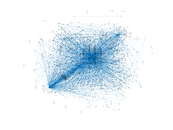### Degree distribution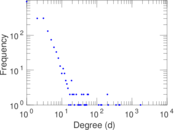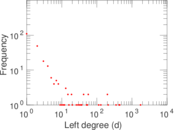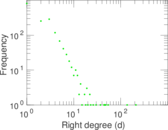### Cumulative degree distribution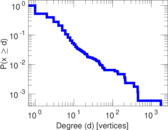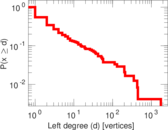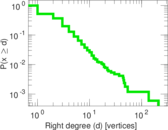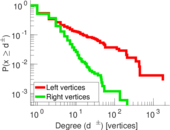### Lorenz curve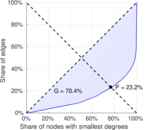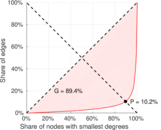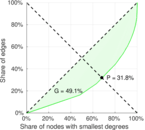### Spectral distribution of the adjacency matrix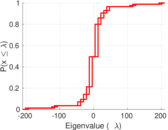### Spectral distribution of the normalized adjacency matrix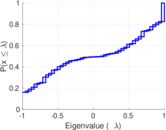### Spectral distribution of the Laplacian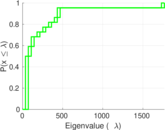### Spectral graph drawing based on the adjacency matrix### Spectral graph drawing based on the Laplacian### Spectral graph drawing based on the normalized adjacency matrix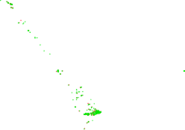### Degree assortativity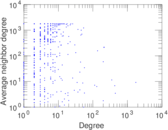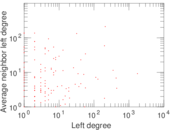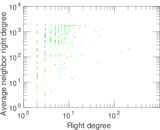### Zipf plot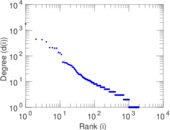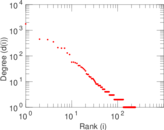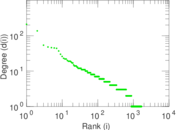### Hop distribution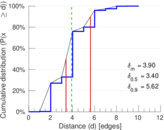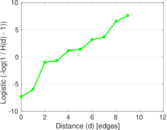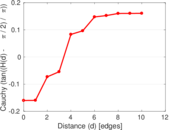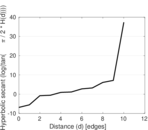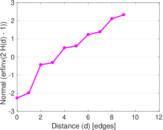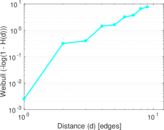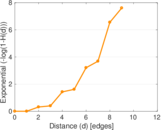### Double Laplacian graph drawing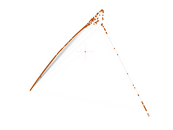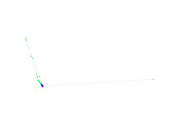### Delaunay graph drawing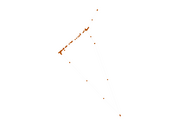### Edge weight/multiplicity distribution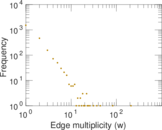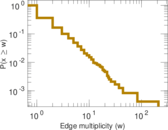### Temporal distribution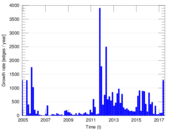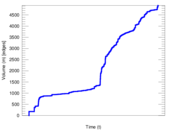### Temporal hop distribution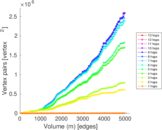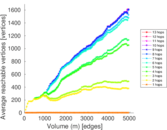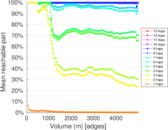### Diameter/density evolution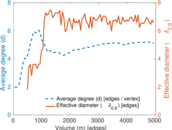### Matrix decompositions plots